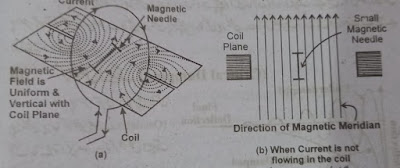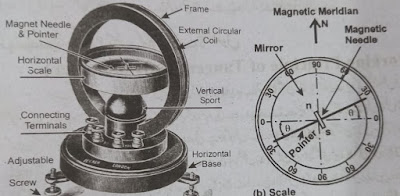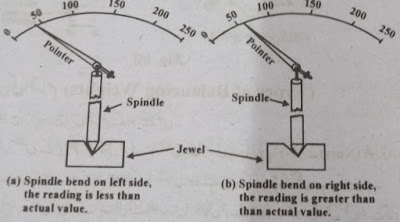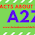# Galvanometer-Working-Construction of Galvanometers-Types-Galvanometer Diagram-Galvanometer Uses

This article provides detailed information about Galvanometers. You can get all the information about the galvanometer from here. The working principle of the galvanometer, theory of galvanometer, parts of the galvanometer, and all uses of galvanometer are explained.

# Galvanometers

A Galvanometer is an absolute electronic device that can accurately measure the amount of current passing through a circuit. It did not need to be calibrated with any other standard device. This meter is also called a moving magnetic meter. The angle at which the pointer of this meter moves is multiplied by the constant Tan of an angle K to find the current passing through the coil of this meter. Therefore it is called a Tangent galvanometer.

## Galvanometer Theory

Suppose there is a circular coil whose number of cycles is "n" and whose radius is 'r'. If an electric current is passed through this coil, the magnitude and direction of the magnetic field formed by it will vary in different places.

The magnetic field in the center of the coil's sphere will be uniform and perpendicular to the plane of the coil. As shown by the shape "a”. If a short length of the magnetic needle is placed in the middle of the coil, so that it can rotate freely on its axis, it will indicate the direction of the magnetic field. When the current is not passing through the coil, this magnetic needle aligns itself with the horizontal component "H" Horizontal of the Earth's magnetic field. This horizontal component is called the Magnetic Meridian. As shown by figure "b". (Remember that the earth itself is a great magnet).Galvanometer Diagram

Now, if the current is passed through the coil, the resulting magnetic field will be perpendicular (90) to the ground magnetic field, as shown by figure "c". The force "F" applied by the coil's magnetic field depends on the following.

F = 2πni / r (Oersted) ........ (1)

In this case, the following two magnetic forces act on this magnetic needle.

1. The magnetic force created by the current passing through the coil
2. The magnetic force generated by the horizontal component H of the ground magnetic field

As a result of the interaction of these two forces, the magnetic needle rotates to a "Ѳ" angle. The value of this force is as follows.

F = H TanѲ……… (2)

### Galvanometer Working Principle

When a magnet is suspended freely in a magnetic field, the magnetic field forces a magnetic force on the bar magnet, forcing it to remain parallel (i.e. in alignment). So a magnetic field is created. Which affects the magnetic needle placed in the middle of the coil. And she tries to keep it aligned. The horizontal component "H" of the earth's magnetic field is already aligned by affecting the magnetic needle (Figure b above). When the two forces on the magnetic needle (which are at 900 on each other) are permeated, the magnetic needle rotates to an angle of "Ѳ".

### Construction/Parts of Galvanometer

The following figure shows the parts of a galvanometer. It has a round plastic frame. In the middle of which there is a groove. In this groove, there are usually three galvanometer coilers consisting of three turns of insulated copper wire. One of these coils consists of just one turn of thick wire.

The second coil bar consists of 50 turns of a single wire while the third coil bar consists of 500 turns of a single wire. Each coil has its own two terminals. A total of six terminals of these three coils are made on the front of the instrument. Sometimes there are four terminals, one of which is common and the other three are used to detect different amounts of current. The size of the coil wire is kept different so that different amounts of current can be measured. For measuring high current, a thick wire with fewer cycles is used and for low current, a single wire coil with more cycles is used.Galvanometers Parts and Construction

The round frame is mounted on a horizontal platform so that the coils (including their terminals) can rotate horizontally at some angles in a circular circle. There is vertical support in the middle of the gum frame. Above which the horizontal scale of the galvanometer is applied in such a way that it falls right in the middle of the coiled round frame. This scale is divided from zero to 900. A short length of the magnetic needle is pivoted in the middle of the scale. A long pointer is usually attached to this magnetic needle in such a way that when the magnetic needle is aligned with the meridian then the pointer is at zero degrees. Sometimes a mirror is also attached to the center of the galvanometer scale. To avoid parallax error, a horizontal platform is formed under the vertical platform. Below are three screwdrivers for leveling the instrument.

### How to use a Galvanometer

The galvanometer is first leveled with the help of adjustable screwdrivers. The frame is then rotated to align the magnetic needle with the ground magnetic meridian. Then, with the help of a Daniel cell, the current is passed through the coils of the frame so that the pointer moves at an angle of less than 90. Now the coil radius "r" is noted from the galvanometer information data plate. The amount of current is determined in amperes by applying the number of angles and angles of the previously known horizontal component "H" in the following formula.

i = 10Hr tanѲ / 2πN.......... in Amperes

### Uses of Galvanometers

Galvanometers are used in standard laboratories for the following purposes:

• For absolute measurement of current
• To measure the horizontal component "H" of the earth's magnetic field at some point.
• For Calibration of Laboratory Standard Instruments
• (Instruments that are used for calibration of common instruments are called laboratory standard instruments.)

#### Importance of balancing the moving system of instruments

The moving system of Spring Control Instruments is attached to a spindle and it is the pivot between the jewels. It is very important to balance the spindle of the moving system. If it is not balanced then the amount of wear on different parts of the Pivot and Jewel will vary due to tilting to one side. This way the jewel will penetrate more from one side. This will cause the spindle to move permanently off its axis of rotation (as shown by the following shapes and b) and the pointer of the instrument will not display the correct reading. That is, the reading will not be reliable. Therefore, the rotating axis of the moving stem of the instrument must be in the exact alignment of the center of gravity for all the positions of the pointer.Galvanometers G

#### See About: Controller Modes

1.1.